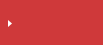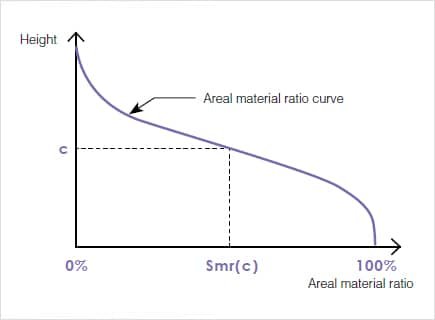>  What is area roughness?  >  Area Roughness Parameters

# Smr(C) (Areal Material Ratio) | Area Roughness Parameters

## surface texture parameters in ISO 25178

• Height
• Spatial
• Hybrid
• Functional
• Functional volume
• FeatureSearch from the parameters list

## Smr(C) (Areal Material Ratio)

The areal material ratio curve of an area is a curve representing heights at which the areal material ratio changes from 0% to 100%.

An areal material ratio represents the area with a specific height c or higher. The areal material ratio at height c corresponds to Smr(c) in the figure below.When a secant line of an areal material ratio curve, which covers 40% of the difference in the areal material ratio, is shifted from the areal material ratio of 0% the position at which the secant line has the smallest gradient is called the center portion of the areal material ratio curve.

The equivalent line is the line where the sum of the squared deviation in the vertical-axis direct is the smallest in the center portion.

The section of the equivalent line between the two height positions where the areal material ratio is 0% and 100% is called the core surface. The peaks with a height above the core surface are called reduces peaks, while the valleys below the core surface are called reduced vallesy.

The core surface represents the height of the area that makes contact with other objects after initial abrasion.## Search from the parameters list

Height Sa (arithmetical mean height) Sz (Maximum height) Sq (Root mean square height) Ssk (Skewness) Sku (Kurtosis) Sp (Maximum peak height) Sv (Maximum pit height) Sal (Auto-correlation length) / Str (Texture aspect ratio) Std* (Texture direction) Sdq (Root mean square gradient) Sdr (Developed interfacial area ratio) Smr(c) Areal material (bearing area) ratio Smc(mr) Inverse areal material ratio Sk(Core height) Spk (Reduced peak height) Svk (reduced dale height (reduced valley depth)) Smr (Peak material portion) Smr2 (Valley material portion) Sxp (Peak extreme height) Vvv (Dale void volume) Vvc (Core void volume) Vmp (Peak material volume) Vmc (Core material volume) Spd (Density of peaks) Spc (Arithmetic mean peak curvature) S10z / S5p / S5v / Sda(c) / Sha(c) / Sdv(c) / Shv(c)